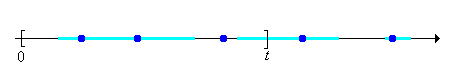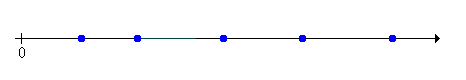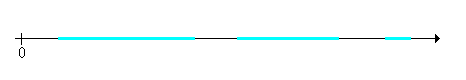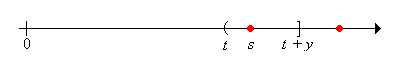]> Renewal Equations

## 2. Renewal Equations

Many quantities of interest in the study of renewal processes can be described by a special type of integral equation known as a renewal equation. Renewal equations almost always arise by conditioning on the time of the first arrival and by using the defining property of a renewal process--the fact that the process restarts at each arrival time, independently of the past. However, before we can study renewal equations, we need to develop some additional concepts and tools involving measures, distribution functions, integrals, convolutions, and transforms. In many ways, these parallel our previous study of probability measures, probability distribution functions, expected value, convolution of probability density functions, and generating functions. Hopefully, these parallels will make the study easier.

### Measures and Integrals

#### Positive Measures and Distribution Functions

Suppose that $G$ is a positive measure (or distribution) on $0$ with the property that $G 0 t$ for each $t 0$ and $G 0 0$ (quite possible infinite). We will also use $G$ to denote the corresponding distribution function:

$G t G 0 t t 0$Hopefully, the notation will not cause confusion and it will be clear from context whether $G$ refers to the measure (a set function) or the distribution function (a point function). The basic structure of a positive measure and its associated distribution function occurred several times in our preliminary discussion of renewal processes:

• The distribution $F$ of the interarrival times
• The counting process $N$ (although this is a random measure).
• the renewal measure $m$

Suppose that $a 0$ and that $G a 0$.

1. Show that the measure $G a$ on $0 a$ defined by $G a A G A G a$ for $A 0 a$ is a probability measure.
2. The corresponding probability distribution function is $G a t G t G a$ for $0 t a$.

The function $G$ satisfies many of the basic properties of a cumulative distribution function. Moreover, the proofs are essentially the same; in fact, the proofs follow from Exercise 1. As usual, we will let $G t s s t G s$ denote the limit of $G$ from the left at $t$. By convention, we will let $G 0 0$

Show that

1. $G$ is nonnegative.
2. $G$ is increasing.
3. $G$ is continuous from the right.
4. $G t G 0 t$ for $t 0$, so in particular, $G$ has limits from the left.
5. $G s t G t G s$ for $0 s t$
6. $G s t G t G s$ for $0 s t$
7. $G s t G t G s$ for $0 s t$
8. $G s t G t G s$ for $0 s t$

A measure on $0$ is completely determined by its values on intervals, so it follows that the measure $G$ is completely determined by the distribution function $G$. Equivalently, a function $G$ that satisfies properties (a), (b), (c), and (d) of Exercise 2 defines a measure through (e), (f), (g), and (h). In almost all cases, the measure $G$ will be discrete, continuous, or mixed, in analogy to the discrete, continuous, and mixed probability distributions that we have studied.

First, $G$ is discrete if there exists a countable set $S 0$ and a function where $g$ from $S$ into $0$ such that

$G A t A S g t , A 0$

Thus, the measure is concentrated at the discrete set of points $S$ and $g t$ is the measure at $t S$. The function $g$ is the density function of the measure $G$ with respect to counting measure.Next, $G$ is continuous if there exists a function $g$ from $0$ into $0$ such that

$G A t A g t , A 0$

Thus, $G$ has no points of positive measure. The function $g$ is the density function of the measure $G$ with respect to standard Lebesgue measure (length measure) on $0$. For our purposes, $g$ will be a nice function that is integrable in the ordinary calculus sense.Finally, $G$ is mixed if it is the sum of a discrete and continuous measure. That is, there exists countable set $S 0$ and a function $g$ from $S$ into $0$ as well as a function $h$ from $0$ into $0$ such that

$G A t A S g t t A h t , A 0$

Thus, part of the measure $G$ is concentrated at the discrete set of points $S$ and the rest of the measure is continuously spread out over $0$.#### Integrals with respect to a Measure

Suppose now that $u$ is a function from $0$ into , and that $A$ is a (measurable) subset of $0$. We will denote the integral of $u$ on the set $A$ with respect to the measure $G$ by

$G x A u x$

We will not go into the technical details of the general definition of this integral. However, in the discrete, continuous, and mixed cases, the integral is very similar to the definitions that we have given for expected value. First, suppose that $G$ is a discrete measure with discrete density $g$ as defined above. Then

$G t A u t t A S u t g t$

Next, suppose that $G$ is a continuous measure with density function $g$ as defined above. Then

$G t A u t t A u t g t$

Finally, suppose that $G$ is a mixed measure with density functions $g$ and $h$, as defined above. Then

$G t A u t t A S u t g t t A u t h t$

This general integral satisfies the essential properties of any integral, which are given in the following exercises. Give proofs at least in the discrete and continuous cases. Assume that $u$ and $v$ are (measurable) functions on $0$, $a$ is a constant, and $A$ is a (measurable) subset of $0$. Assume also that the indicated integrals exist.

The integral is a linear operation:

1. $G t A u t v t G t A u t G t A v t$
2. $G t A a u t a G t A u t$

The integral is a monotone operator:

1. If $u$ is nonnegative on $A$ then $G t A u t 0$
2. If $u t v t$ for $t A$ then $G t A u t G t A v t$

In the remainder of this section, unless otherwise noted, we will denote the integral over the closed interval $0 t$ by

$G s 0 t u s$

#### Convolution

As above, suppose that $G$ is a positive measure on $0$ and that $u$ is a function from $0$ into . The convolution of the function $u$ with the distribution $G$ is the function $u G$ defined by

$u G t G s 0 t u t s$

This is a different use of the word than in our previous study of the convolution of probability density functions, but there is a close connection.

Suppose that $G$ is a probability distribution with density function $g$ and that $u$ is a probability density function (either both discrete or both continuous). Show that $u G u g$ where the convolution on the right is the ordinary convolution of probability density functions. Recall that this function is the probability density function of the sum of two independent random variables, one with probability density function $g$ and one with probability density function $u$.

Convolution is associative. Suppose that $G$ and $H$ are measures on $0$ and that $u$ is a function on $0$. Show that

$u G H u G H$

Convolution is linear. Suppose that $G$ is a measure on $0$, that $u$ and $v$ are functions on $0$, and that $c$ is a constant. Show that

1. $u v G u G v G$
2. $c u G c u G >$

In general, the commutative property does not make sense since the function $u$ and the measure $G$ are different types of objects. In the special case that the function is the cumulative function of a measure, the commutative property does hold.

Suppose that $G$ and $H$ are measures on $0$. Show that

$G H H G$

### Renewal Equations and Their Solutions

Armed with our new analytic machinery, we can return to the study of renewal processes. Thus, suppose that we have a renewal process with interarrival distribution function $F$ and renewal function $m$. Recall that each of these functions defines a positive measure on $0$, as discussed above. Of course, the measure associated with $F$ is actually a probability measure.

The distributions of the arrival times are the convolution powers of $F$. Show that $F n F F F F n$.

Suppose now that $a$ and $u$ are functions on $0$, with $a$ known and $u$ unknown. An integral equation of the form

$u a u F$

is called a renewal equation for $u$. Usually, $u t U t$ where $U t t 0$ is a random process of interest associated with the renewal process. The renewal equation comes from conditioning on the first arrival time $T 1 X 1$, and then using the defining property of the renewal process--the fact that the process starts over, interdependently of the past, at the arrival time.

Condition on the first arrival time to show that $m F m F$.

Thus, the renewal function itself satisfies a renewal equation. Of course, we already have a formula for $m$, namely $m n 1 F n$. However, sometimes $m$ can be computed more easily from the renewal equation directly.

The following exercises give the fundamental results on the solution of the renewal equation.

Show that $u a a m$ is a solution of the renewal equation $u a u F$. Moreover, show that if $a$ is locally bounded, then $u$ is locally bounded and is the unique such solution. The steps are given below.

1. Show that $u F a F a m F a F a m F a m u a$ and conclude the $u$ is a solution.
2. Suppose that $a s C t$ for $0 s t$. Show that $u s 1 m t C t$ for $0 s t$.
3. Continuing, suppose that $v$ is another locally bounded solution of the integral equation, and let $w u v$. Show that $w$ is locally bounded.
4. Show that $w w F$.
5. Show that $w w F n$ for $n$.
6. Suppose that $w s D t$ for $0 s t$. Show that $w t D t F n t$ for $n$
7. Since $m t$ it follows that $F n t 0$ as $n$.
8. Conclude that $w t 0$.

#### The Distribution of the Age Variables

Let $R t$ denote the remaining life at time $t$ and for $y 0$, let

$u y t R t y N t t y 0$

We will derive and then solve a renewal equation for $u y$ by conditioning on the time of the first arrival. We can then find integral equations that describe the distribution of the current age and the joint distribution of the current and remaining ages.

Show that

1. $R t y X 1 s R t s y$ for $s 0 t$2. $R t y X 1 s 0$ for $s t t y$3. $R t y X 1 s 1$ for $s t y$Now let $F t 1 F t X t$ for $t 0$ (the right-tail distribution function of an interarrival time), and for $y 0$, let $F y t F t y$

Condition on the time of the first arrival and use the result of the previous exercise to show that $u y$ satisfies the renewal equation

$u y F y u y F$

Solve the renewal equation to show that

$R t y F t y m s 0 t F t y s , y 0$

Now let $C t$ denote the current age at time $t$ and recall that $C t x R t x x$ for $0 x t$. Use this result and the Exercise 14 to show that

$C t x F t m s 0 t x F t s , 0 x t$

Next recall that $C t x R t y R t x x y$ for $y 0$ and $0 x t$. Use this result and Exercise 14 to show that

$C t x R t y F t y m s 0 t x F t y s , 0 x t , y 0$

### Examples and Special Cases

#### Uniformly Distributed Interarrivals

Consider the renewal process with interarrival times uniformly distributed on $0 1$. Thus the probability distribution function of an interarrival time is $F x x$ for $0 x 1$. The renewal function $m$ can be computed from the renewal equation in Exercise 10 by successively solving differential equations.

Show that $m t t 1$ for $0 t 1$:

1. In the integral in the renewal equation, use the substitution $y t s$
2. Differentiate the equation in (a) with respect to $t$ to show that $m$ satisfies the differential equation $m t 1 m t$ for $0 t 1$
3. Solve the differential equation in part (b) subject to the initial condition $m 0 0$.

Show that $m t t 1 t 1 t 1$ for $1 t 2$:

1. In the integral in the renewal equation, use the substitution $y t s$.
2. Show that $m$ satisfies the differential equation $m t 1 t 1 m t$ for $1 t 2$
3. Solve the differential equation in (b) subject to initial condition $m 1 1$

#### The Poisson Process

Recall that the Poisson process has interarrival times that are exponentially distributed with rate parameter $r 0$. Thus, the interarrival distribution function is $F x 1 r x$ for $x 0$.

Use the renewal equation in Exercise 10 to give another proof that the renewal function is $m t r t$ for $t 0$.

1. In the integral in the renewal equation, use the substitution $y t s$
2. Differentiate the result in part (a) with respect to $t$ to show that $m$ satisfies the differential equation $m t r$ for $t 0$.
3. Solve the differential equation in part (b) with the initial condition $m 0 0$

Use the result of Exercise 16 to give another derivation of the joint distribution of $C t R t$.

1. $C t$ and $R t$ are independent.
2. $R t$ has the same distribution as an interarrival time, namely the exponential distribution with rate parameter $r$>.
3. The current life at time $t$ has a truncated exponential distribution with parameters $t$ and $r$: $C t s r s 0 s t 0 s t$

#### Bernoulli Trials

Consider the renewal process for which the interarrival times have the geometric distribution with parameter $p$:

$f n 1 p n 1 p , n$

The arrivals are the successes in a sequence of Bernoulli trials. The number of successes $Y n$ in the first $n$ trials is the counting variable for $n$.

Use the renewal equation in Exercise 10 to give another proof that the renewal function is $m n n p$ for $n$.

Use the result of Exercise 16 to give another derivation of the joint distribution of $C n R n$ for $n$

1. $C n$ and $R n$ are independent.
2. $R n$ has the same distribution as an interarrival time, namely the geometric distribution with parameter $p$.
3. $C n$ has a truncated geometric distribution with parameters $n$ and $p$: $C n k p 1 p k k 0 1 n 1 1 p n k n$